# QlikView App Dev

Discussion Board for collaboration related to QlikView App Development.

cancel
Showing results for
Did you mean: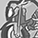Contributor II

## Evaluate surrounding rows

Hi,

given e.g. a table:

IDValue
1A
2B
3B
4A
5B

I want to be able to evaluate the surrounding rows of every row.

That means for example to get an expression that results in this additional column:

magic
0
1
2
0
1

It tells, how many of the identical values surround the current row up to 2 rows in each direction.

1: is A -> no A in 2 or 3 => 0

2: is B -> no B in 1, B in 3, no B in 4 => 1

3: is B -> no B in 1, B in 2, no B in 4, B in 5 => 2

I want to be able to do this and similar questions.

Another question would be: How many rows with the same value are (sequentially) above me/below me?

resulting in (for above): 0, 0, 1, 0, 0 - because just the B in 3 has another B above.

1 Solution

Accepted SolutionsMVP

Hi,

maybe one dynamic solution might be: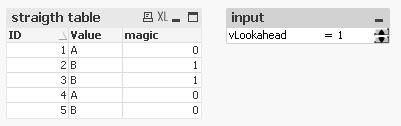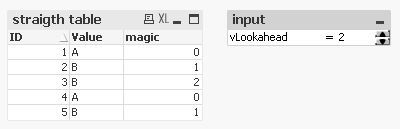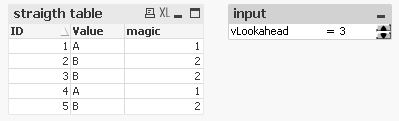using this 'magic' expression:

hope this helps

regards

Marco

15 RepliesMVP

Data:

Value

FROM table;

New:

Value,

rowno() as Row

Resident Data

order by ID;

drop table Data;

left join(New)

1 as Flag

Resident Data

where Row=1;

Final:

if(Flag=1,0,rangesum(peek(Magic),1)) as Magic

resident New;

drop table New;Contributor II
Author

I'm sorry - I wasn't clear enough.

I want this without the script. Only expressions in a straight table.

I want to be able to e.g. set the number of rows as a variable, changeable by the user.MVP

I am not sure about this set the number of rows as a variable, changeable by the user.

what do you mean by this? Can you please give one example with what exactly you want to achieveContributor II
Author

In my original post I wrote "surround the current row up to 2 rows in each direction"

Now I want that this number - 2 - is stored in a variable and can be change by the user via an input field.

This is not a critical reqirement anyway, because I can just use \$(vLookahead) instead of 2.

More important is the requirement that is is not in the load-script, but in an expression.

I want to be able to highlight a cell, if the current cell is surrounded by more than e.g. 3 rows with the same value.

An this should work also, when the user changes the order of the table.MVP

May be like this?

RangeCount(

If(Value=Above(Value),1),

If(Value=Above(Value,2),1),

if(Value=Below(Value),1),

if(Value=Below(Value,2),1)

)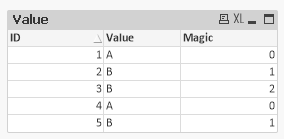MVP

A simpler expression without IFs:

Fabs(

RangeSum(

Value=Above(Value),

Value=Above(Value,2),

Value=Below(Value),

Value=Below(Value,2)

)

)Contributor II
Author

That's pretty much on the path where I am now.

however, now there comes the requirement that I want the 2 be variable (see my answer to kush141087‌)MVP

I don't think this will be achievable from front end because input field function only works from script.MVP & Luminary